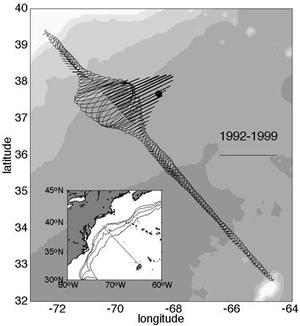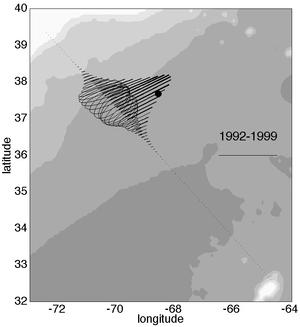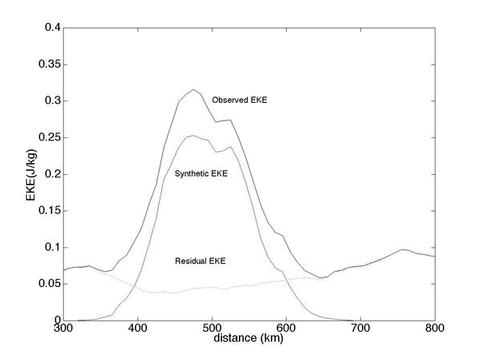Meandering versus mesoscale eddies

Most of the variance in the Eulerian average comes from the meandering of the structurally invariant current.

This is shown in two ways.

Approach 1

Take the observed positions and directions of maximum flow for each and every section and replace all data with data using the analytical form (light line) shown in Figure 2 after projecting them onto the ship's track.
 Eulerian mean Resulting mean field and variance ellipses.Figure 1. The Eulerian mean field and variance ellipses at 52 m depth from >300 sections across the NW Atlantic Figure 3: Synthesis of the Eulerian Gulf Stream statistics by mapping the exponential fit (light line) in Figure 2 back onto the Oleander track. Only the position and direction of the maximum velocity vector are used to orient the double exponential function.

• The similarity with the observed Eulerian mean fields in Figure 1 is striking.
• The velocities are almost identical as are the shapes and orientations of the variance ellipses.
Approach 2

Compare the eddy statistics for the two cases, the original data set (solid line) and the synthetic data set (dashed line).

Define a 'residual eddy kinetic energy' (rEKE) by subtracting the analytical representation of the Gulf Stream from the observations (dotted line) in Figure 4 (below).Figure 4. The three curves show the velocity variance in 1) stream coordinates from the observed data, 2) using only the light line double-exponential form in Figure 2, and 3) after first removing the same double exponential function from each transect. The abscissa shows distance in km from New Jersey.
• 80% of the observed EKE in the center of the current can be accounted for by the meandering of a rigid stream.
• But the difference in peak EKE between the observed and synthetic EKE, while comparable, is actually less than the general EKE level to the sides of the Gulf Stream (0.07-0.09 J/kg).
• The rEKE, which measures the departures of the observed velocity field from the double-exponential form, remains nearly invariant across the entire region, but it too evinces a clear minimum at the center of the current indicating less departure from the 'ideal' form at the center than anywhere else.
In summary, here as at the Pegasus line, most of the observed variance in the region of the Gulf Stream stems from the meandering of the current itself, about 67% of the total between 0 and 2000 m at the Pegasus line (HR) and 80% along the Oleander line at 52 m depth.